# Frequency Distribution

Frequency Distribution

## Table of Contents

 1 Introduction to Frequency Distribution 2 What is Frequency Distribution? 3 Understanding Frequency Distribution 4 Frequency Distribution Table and Examples 5 What is Frequency Distribution with Example 6 Thinking out of the Box! 7 Types of Frequency Distribution 8 Important Notes on Frequency Distribution 9 Practice Questions on Frequency Distribution 10 Maths Olympiad Sample Papers 11 Frequently Asked Questions (FAQs)

We at Cuemath believe that Math is a life skill. Our Math Experts focus on the “Why” behind the “What.” Students can explore from a huge range of interactive worksheets, visuals, simulations, practice tests, and more to understand a concept in depth.

Book a FREE trial class today! and experience Cuemath’s LIVE Online Class with your child.

## Introduction to Frequency Distribution

In our day-to-day lives, we come across a lot of information in the form of numerical figures, tables, graphs, etc.

This information could be marks scored by students, temperatures of different cities, points scored in matches, etc.

The information that is collected is called data.

Once the data is collected, we have to represent it in a meaningful manner so that it can be easily understood.

The data should be organised in such a way that all its features are summarized in a table.

This will help us understand all the features just by a glance.

So, how do we go about doing this?

Well, frequency distribution is one of the ways to organise data.

We will learn all about it here.

## What is Frequency Distribution?

### Frequency Distribution: Definition

In statistics, frequency refers to the number of times a value occurs.

Thus, frequency distribution refers to a table that shows an item and its frequency.

Let's consider an example to understand this better.

A school conducted a blood donation camp.

The blood groups of 30 students were recorded as follows.We can represent this data in a tabular form.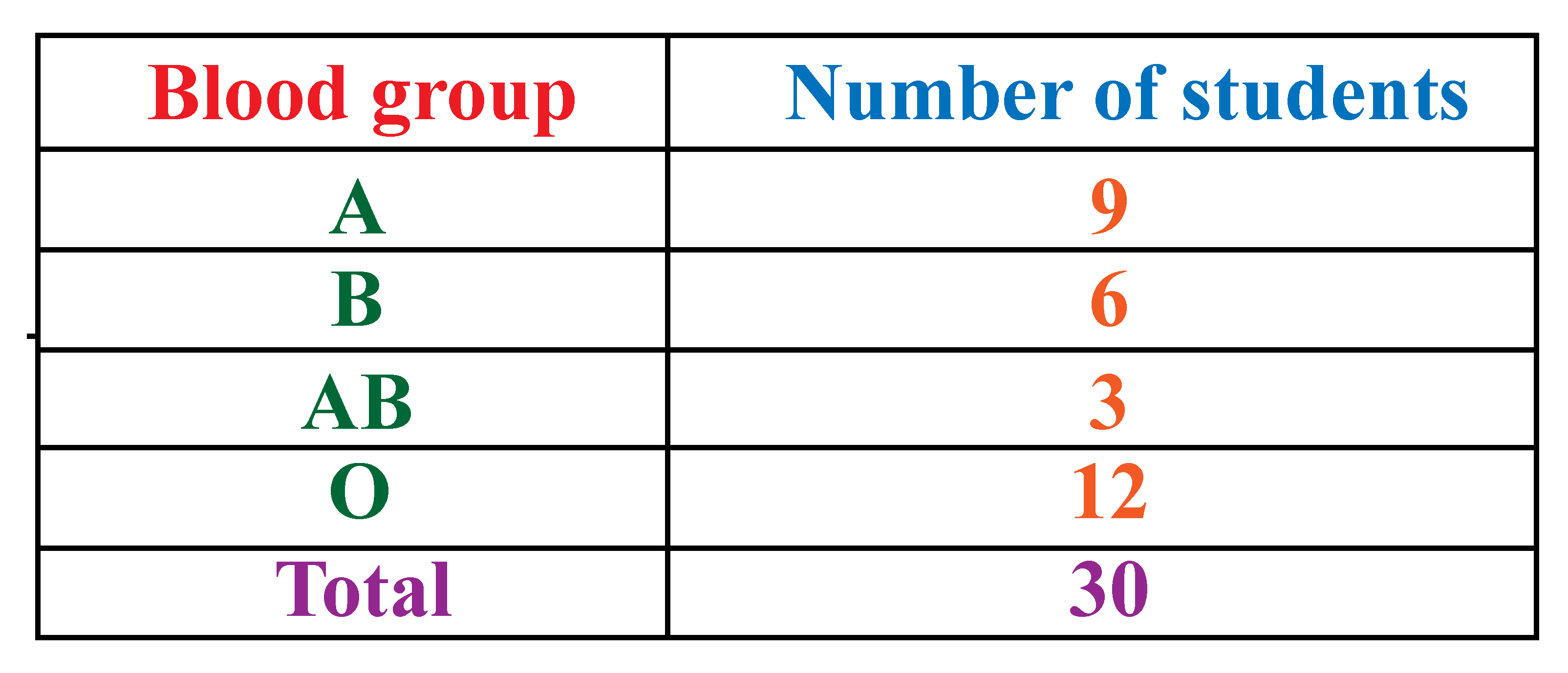This table is known as a frequency distribution table.

You can observe that all the collected data is organised under two columns.

This makes it easy for us to understand the given information.

Thus, frequency distribution in statistics helps us to condense the data in a simpler form so that it is easy for us to observe its features at a glance.

## Understanding Frequency Distribution

### Frequency Distribution in Statistics

Now, let us look at a few examples where we can use frequency distribution tables.

Meet Ms. Jolly and her assistant Mr. Thomas.

Ms. Jolly is a teacher.

She wants to look at the marks obtained by her students in the last exam.

She does not have the time to go through each test paper individually to see the marks.

Hence, she asks Mr. Thomas to organise the data in a table so that it is easier for her to look at all the marks.Ms. Jolly suggests using a frequency distribution table to organise the data.

Using a frequency distribution table here is a good way to present the data as it will show Ms. Jolly all the students' marks in one table.

Mr. Thomas works hard to put together all the data.

He places the marks into certain categories like highest marks, lowest marks, and so on.Let's consider another example.

Consider this jar containing beads of different colours.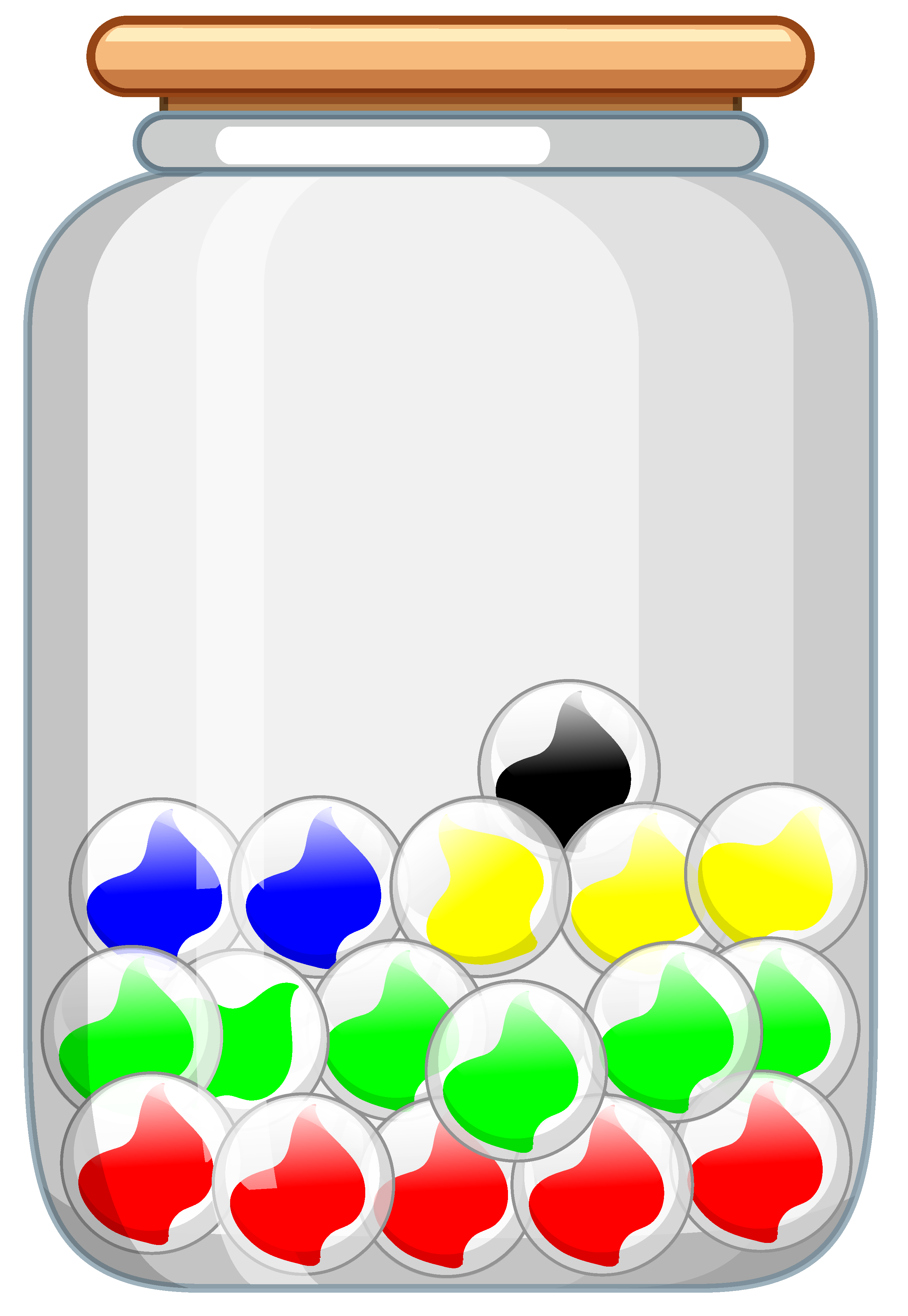To know the exact number of beads of each particular colour, we need to classify the beads into categories.

An easy way to find the number of beads of each colour is to use tally marks.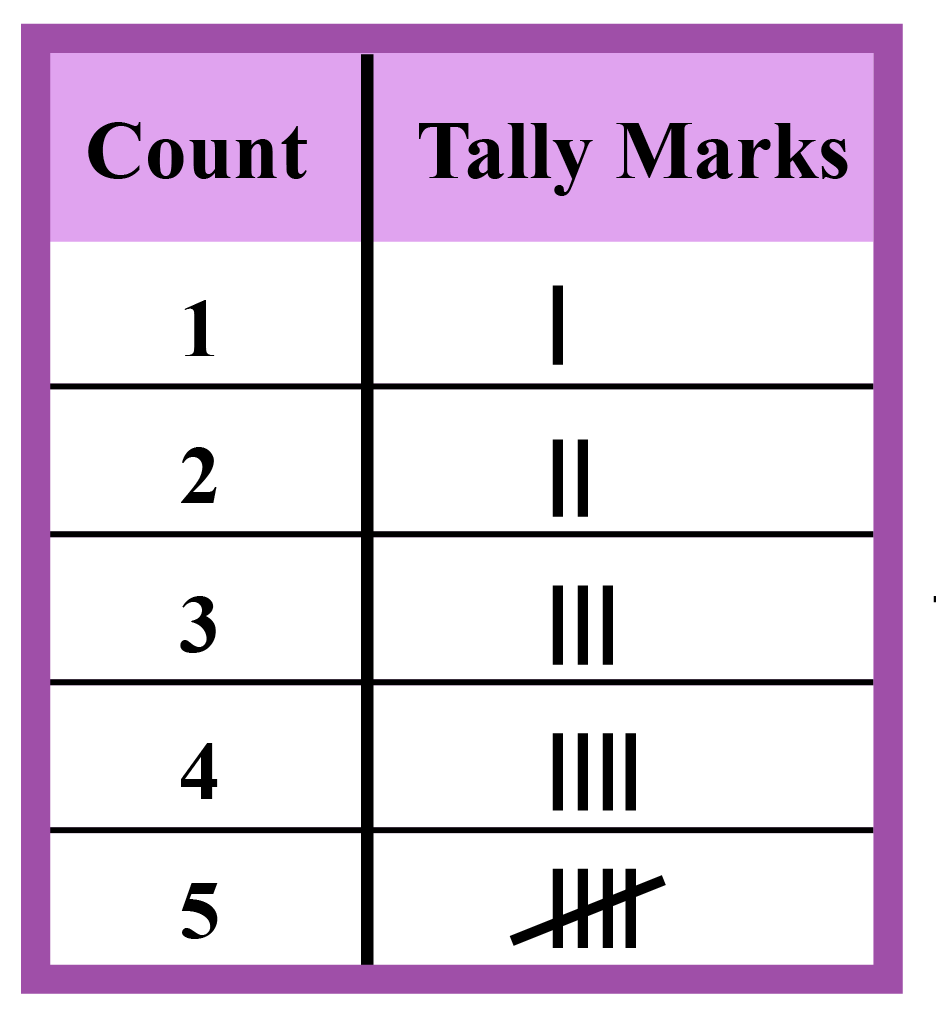Remember that a diagonal line across 4 vertical lines counts as 5

Now, pick the beads from the jar one by one and show the tally marks in the respective table.

Then, indicate the frequency for each item in the table.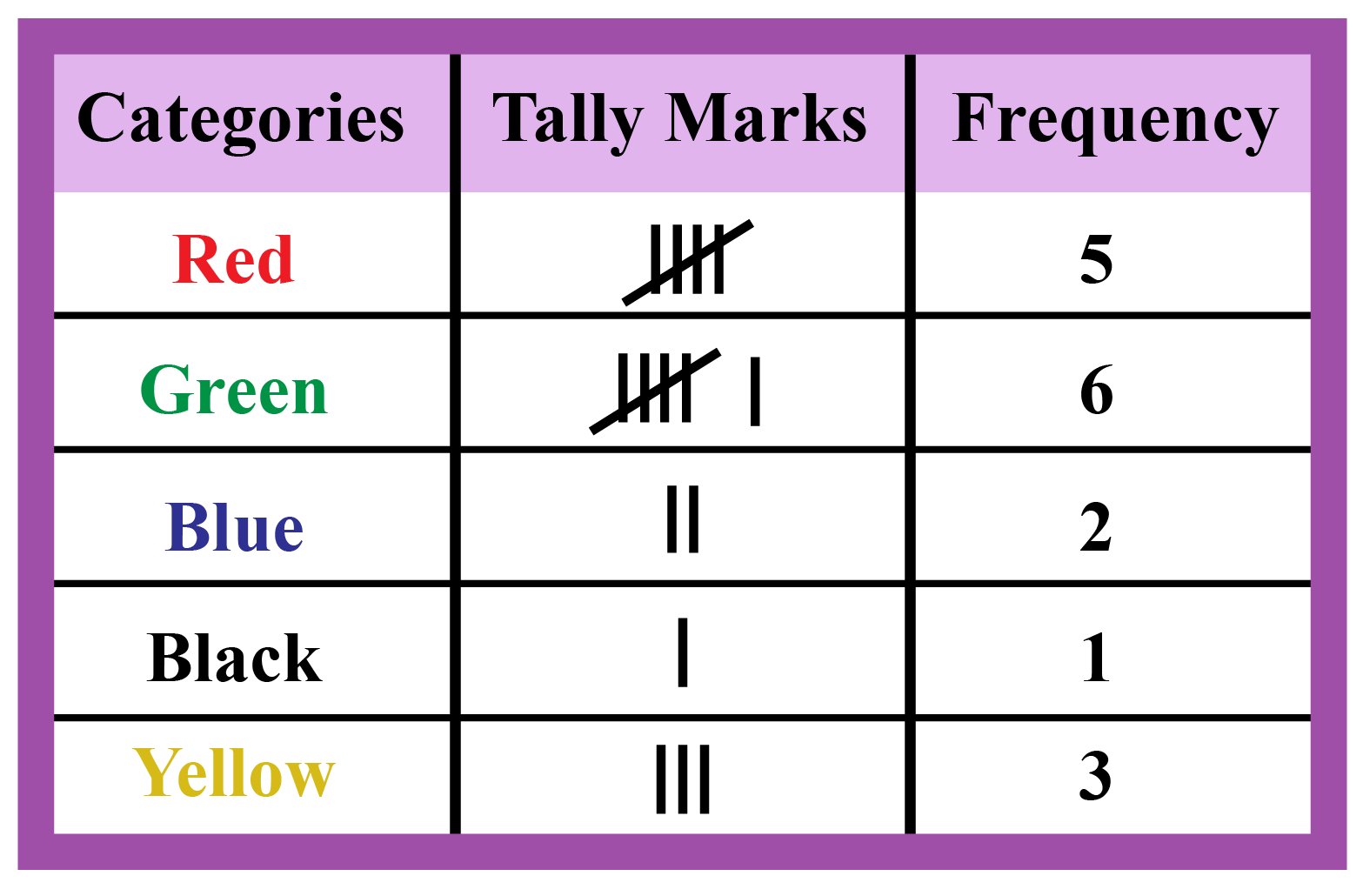Thus, the table so obtained is called a frequency distribution table.

Help your child score higher with Cuemath’s proprietary FREE Diagnostic Test. Get access to detailed reports, customised learning plans and a FREE counselling session. Attempt the test now.

## Frequency Distribution Table and Examples

 Example 1

There are 20 students in a class.

The teacher, Ms. Jolly, asked the students to tell their favourite subject.The results are listed below.

Mathematics English Science
Science Mathematics Science
English Art Mathematics
Mathematics Science Art
Art Science Mathematics
Art Mathematics English
English Mathematics

By looking at this list, can you identify the most-liked subject and the least-liked one?

### Solution:

Since the data is not organised, it is hard to answer this question by just looking at the above data.

To answer this question, let's organise the given data first.

We have four subjects.

20 students have indicated their choices of preferred subjects.

Let us represent this data using tally marks.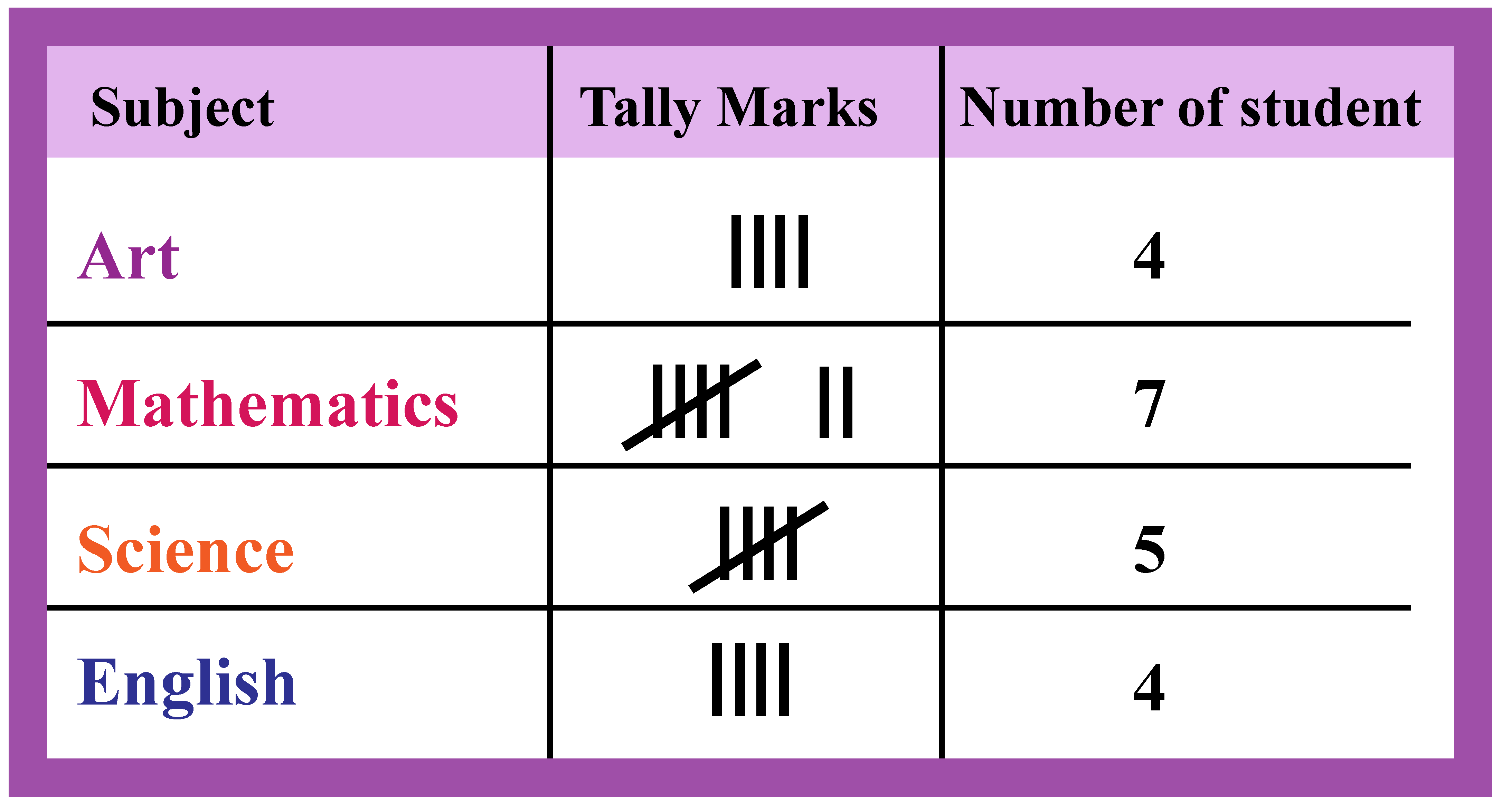The table shown above is called a frequency distribution table.

The tally marks show the frequency of each subject.

In other words, the number of tallies for each subject shows the number of students who like that particular subject.

 $$\therefore$$ Most-liked subject: Mathematics Least-liked subjects: English and Art.
 Example 2

On World Environment Day, 100 schools decided to plant 100 tree saplings in their gardens.The following data shows the number of plants that survived in each school after one month.

95 67 28 32 65 65 69 33 98 96
76 42 32 38 42 40 40 69 95 92
75 83 76 83 85 62 37 65 63 42
89 65 73 81 49 52 64 76 83 92
93 68 52 79 81 83 59 82 75 82
86 90 44 62 31 36 38 42 39 83
87 56 58 23 35 76 83 85 30 68
69 83 86 43 45 39 83 75 66 83
92 75 89 66 91 27 88 89 93 42
53 69 90 55 66 49 52 83 34 36

Using this data, can you find the number of schools that were able to retain 50% of the plants or more?

### Solution:

We need to represent this large amount of data in such a way that a reader can understand it easily.

To include all the observations in groups, we will create various groups of equal intervals.

These intervals are called class intervals.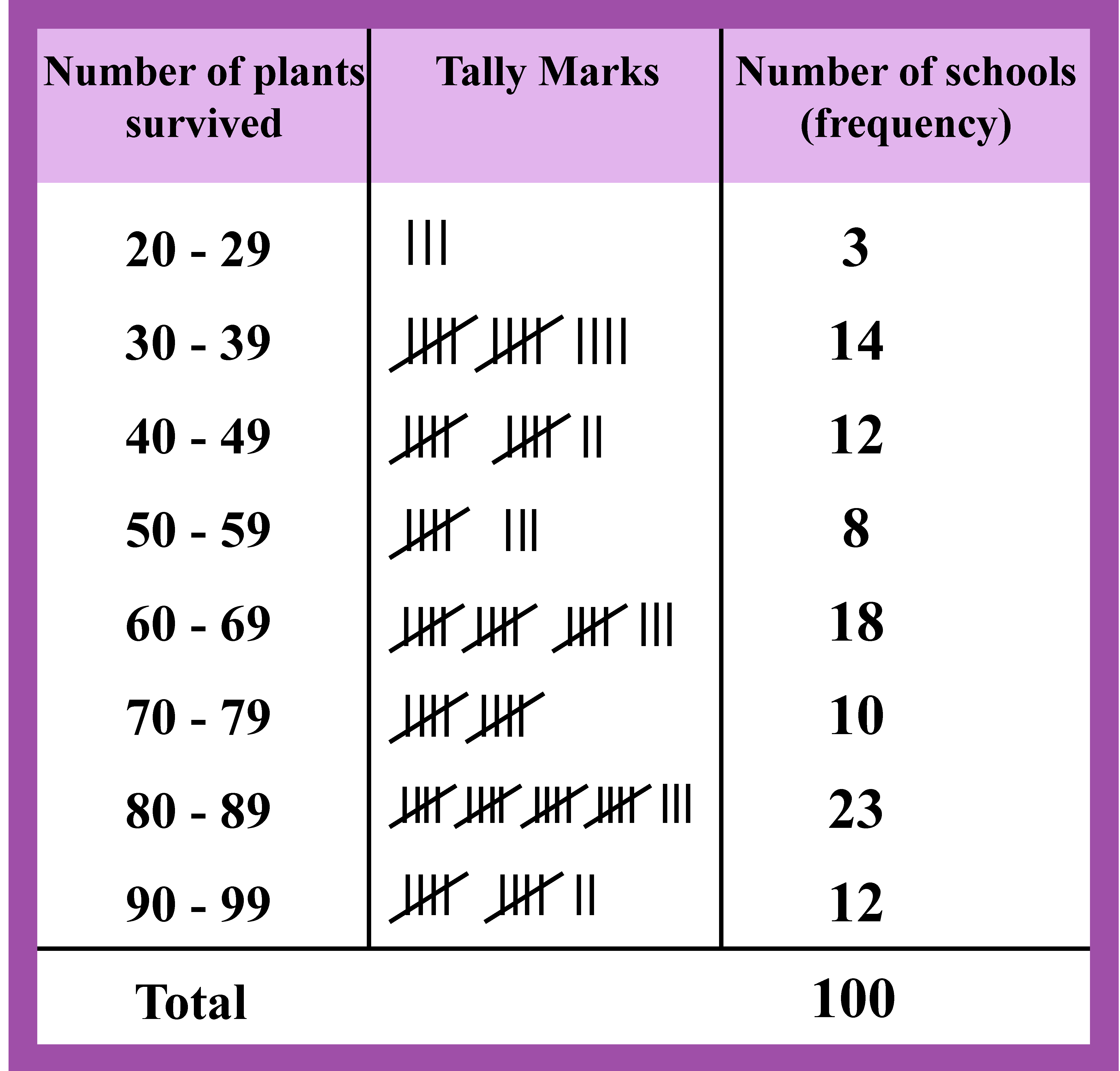From this table, it is clear that 50% or more plants survived in (8 + 18 + 10 + 23 + 12) schools.

 $$\therefore$$ 71 schools were able to retain 50% or more plants in their garden.

## What is Frequency Distribution with Example

The representation of data with tables has already been discussed.

Use the simulation given below to practice frequency charts for different data.

Now let us look at another way to represent data i.e., graphical representation of data.

This is done using a Frequency Distribution Graph.

Such graphs make it easier to understand the collected data.

The graphical representation of data can be shown using the following:

### Bar Graphs

Bar graphs represent data using bars of uniform width with equal spacing between them.

The following bar graph shows the ages of teachers in a school.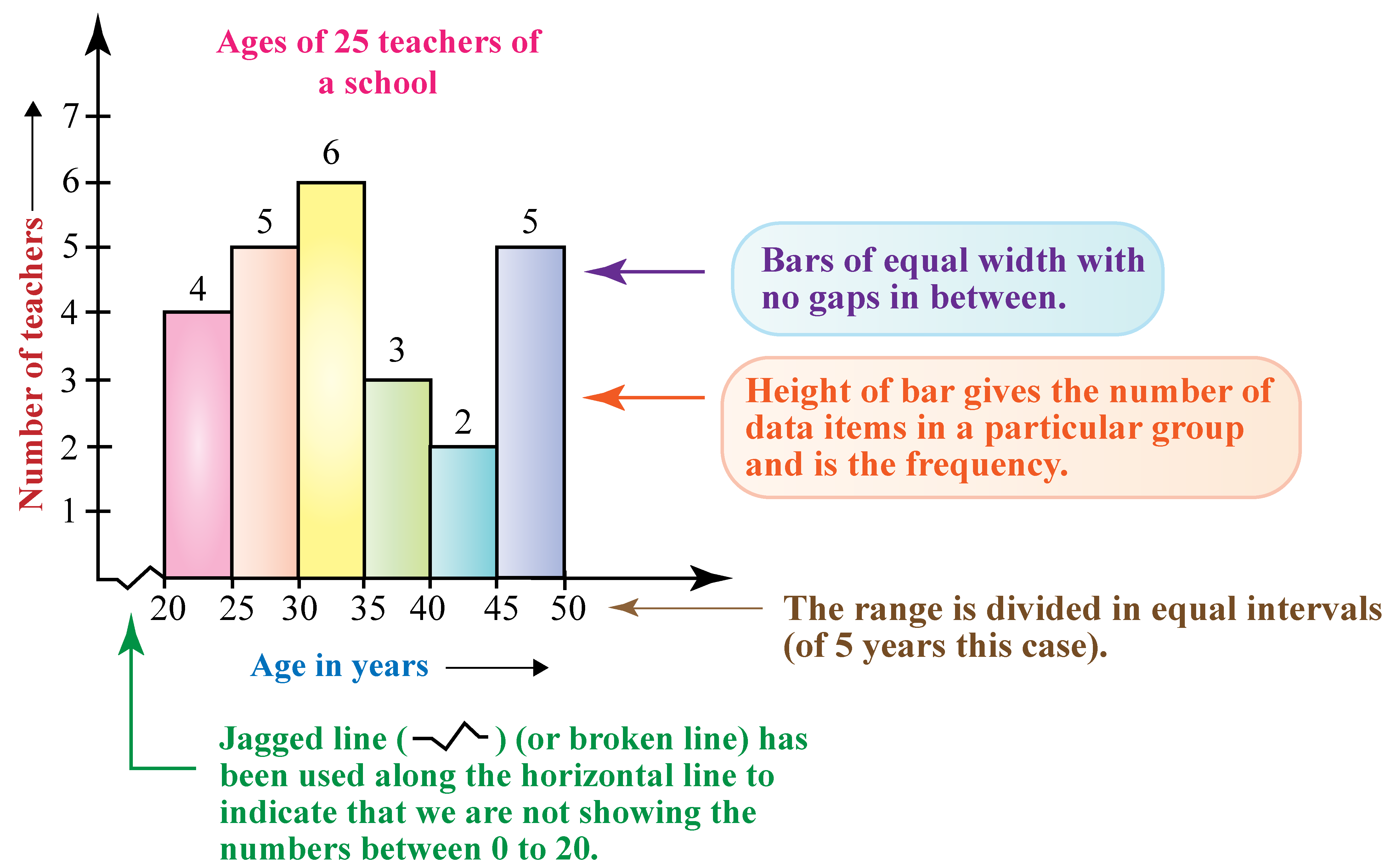### Pie Charts

A pie chart shows a whole circle divided into sectors where each sector is proportional to the information it represents.

The following pie chart shows the time spent by a child for certain activities during a day.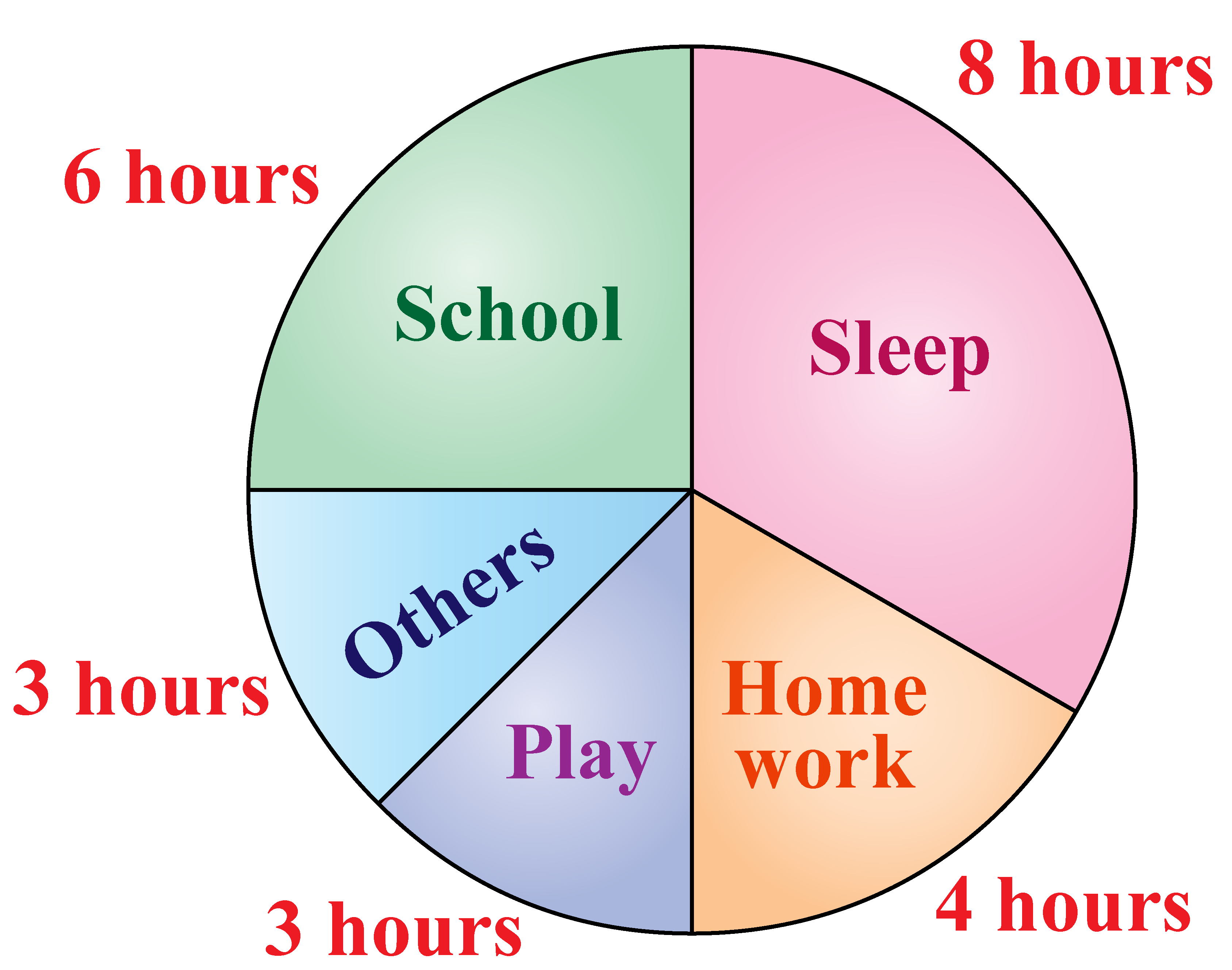### Frequency Polygon

A frequency polygon is drawn by joining the mid-points of the bars in a histogram.

The following frequency distribution graph shows the weights of students in a school.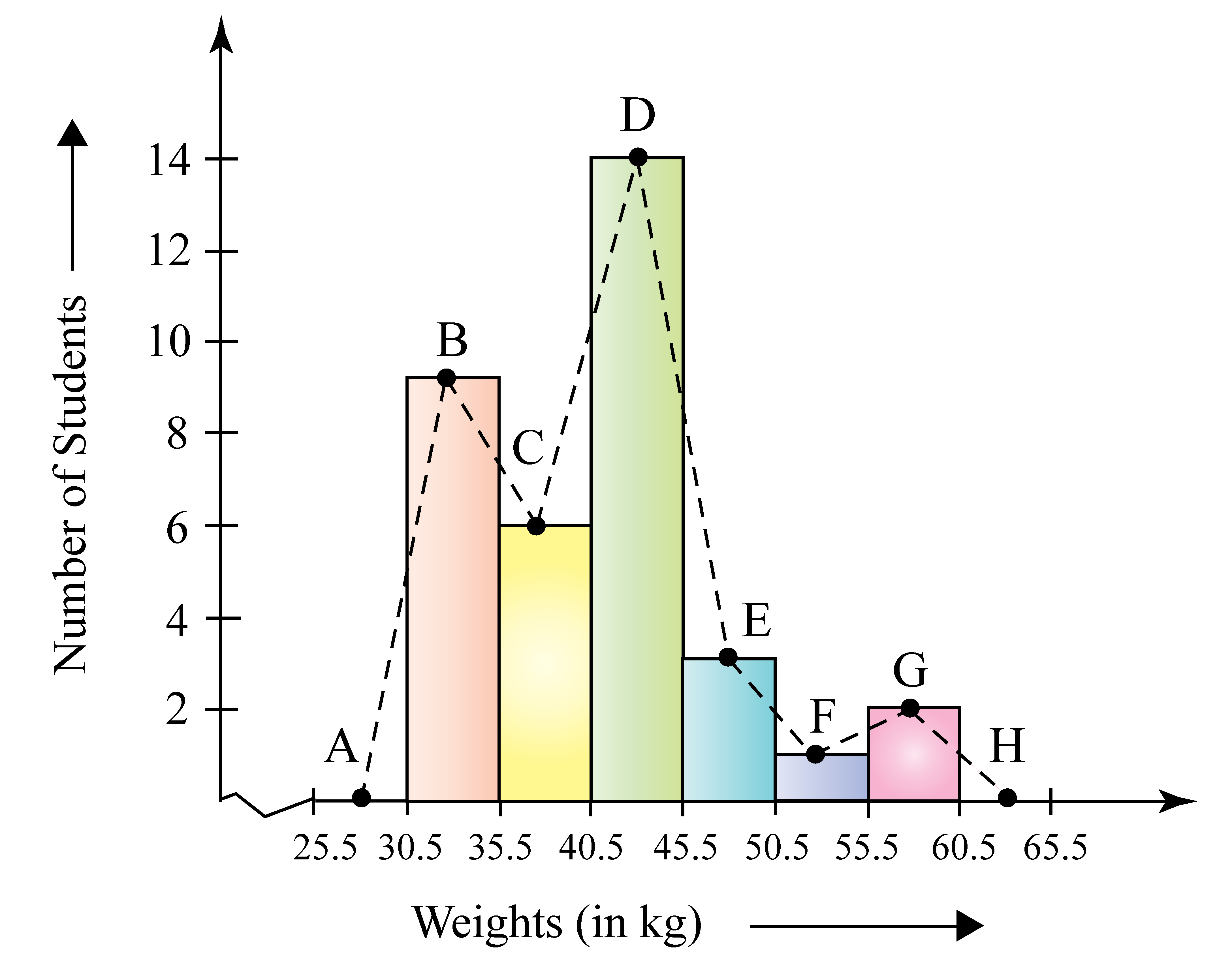Think Tank
 1. The following pie chart shows the data for the modes of transport to school.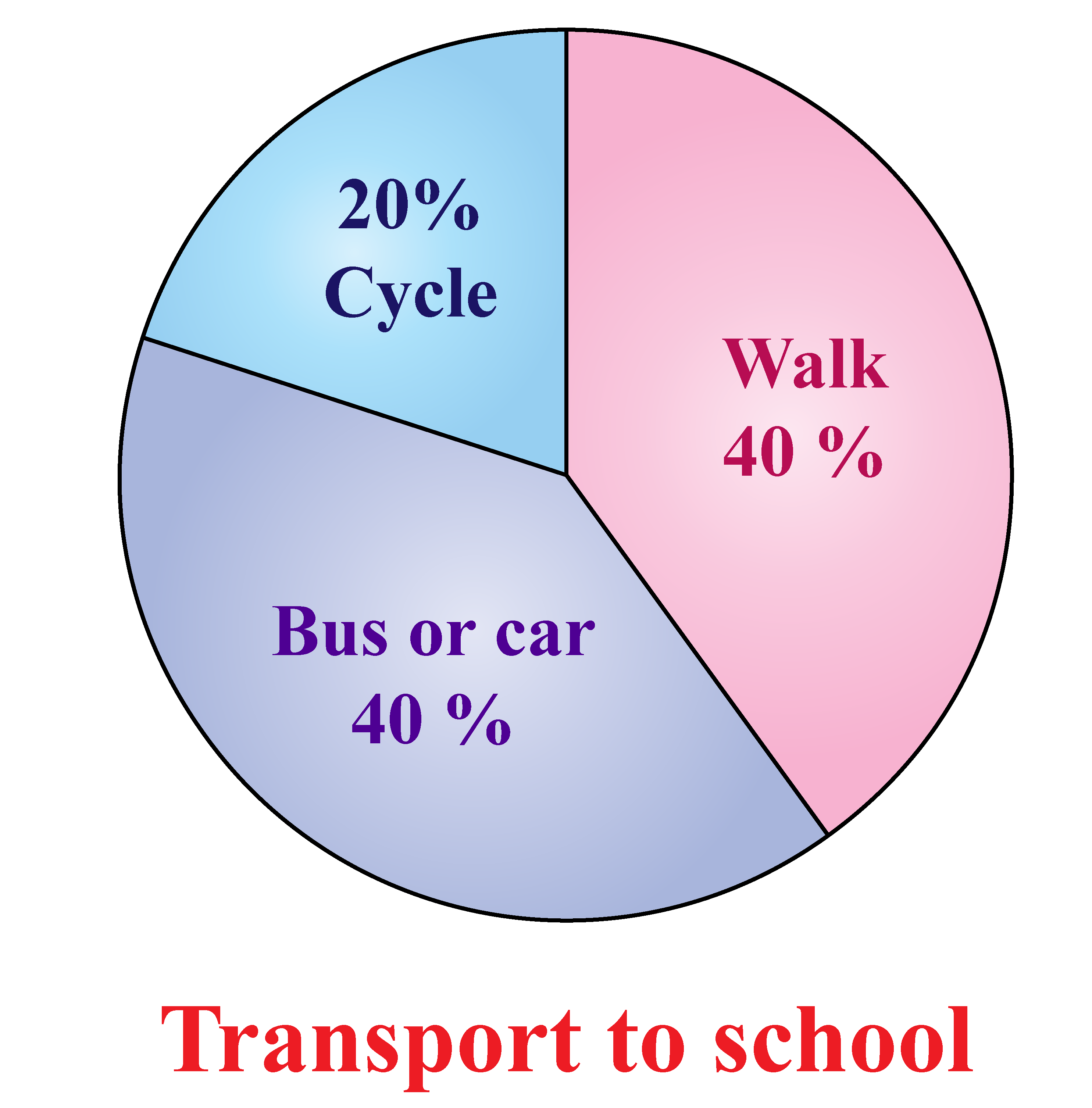Can you write the fraction for representing each of the information given in the pie chart?

## Types of Frequency Distribution

There are two types of frequency distribution tables.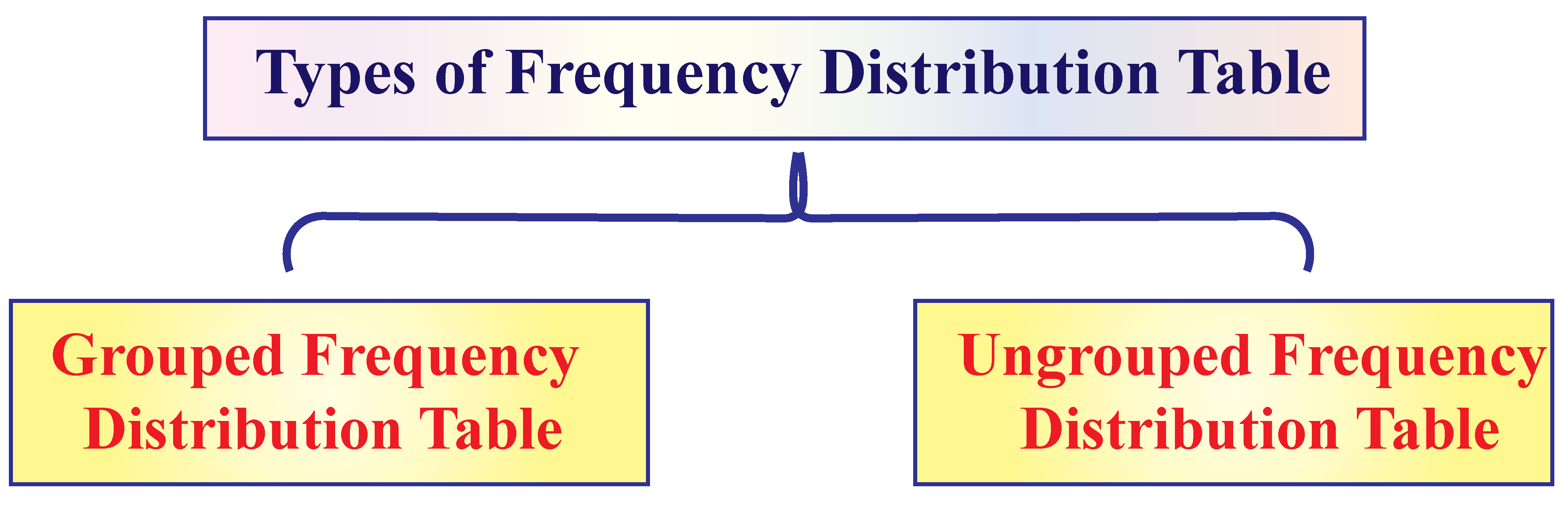### Grouped Frequency Distribution Table

Imagine how difficult it would be if you have a large number of observations.

The table we will obtain will be quite lengthy and the data will be confusing.

Hence, in such cases, we form class intervals to tally the frequency for the data that belongs to that specific class interval.

Marks obtained in the test Number of students (Frequency)
0 - 5 3
5 - 10 11
10 - 15 38
15 - 20 34
20 - 25 9
25 - 30 5
Total 100

A frequency distribution table such as the one above is called a grouped frequency distribution table.

### Ungrouped Frequency Distribution Table

This is used for smaller data sets.

The following table shows the test scores of 20 students.

Marks obtained in the test Number of students (Frequency)
9 1
11 4
13 1
18 1
20 1
21 2
22 1
23 3
25 1
26 3
29 1
30 1
Total 20

A frequency distribution table such as the one above is called an ungrouped frequency distribution table.

Want to understand the “Why” behind the “What”? Explore Frequency Distribution with our Math Experts in Cuemath’s LIVE, Personalised and Interactive Online Classes.

Make your kid a Math Expert, Book a FREE trial class today!

## Practice Questions

Here are few activities for you to practice.

Select/Type your answer and click the "Check Answer" button to see the result.

## Maths Olympiad Sample Papers

IMO (International Maths Olympiad) is a competitive exam in Mathematics conducted annually for school students. It encourages children to develop their math solving skills from a competition perspective.

You can download the FREE grade-wise sample papers from below:

To know more about the Maths Olympiad you can click here

## 1. What is frequency distribution in statistics?

Frequency distribution is a tabular or graphical representation of the data that shows the frequency of all the observations.

## 2. How do you find frequency distribution?

To make a frequency chart, write the categories in the first column.

Next, tally the score in the second column.

Finally, count the tally to write the frequency of each category in the third column.

## 3. What are the 3 types of frequency distributions?

The 3 types of frequency distributions are:

1. Ungrouped Frequency Distribution
2. Grouped Frequency Distribution
3. Relative Frequency Distribution

## 4. Why are frequency distributions important?

Frequency charts are the best way to organize data.

Doctors use it to understand the frequency of diseases.

Sports analysts use it to understand the performance of a sportsperson.

Wherever you have a large amount of data, frequency distribution makes it easy to analyse this data.

More Important Topics
Numbers
Algebra
Geometry
Measurement
Money
Data
Trigonometry
Calculus